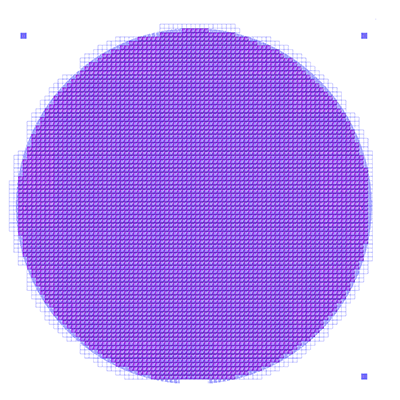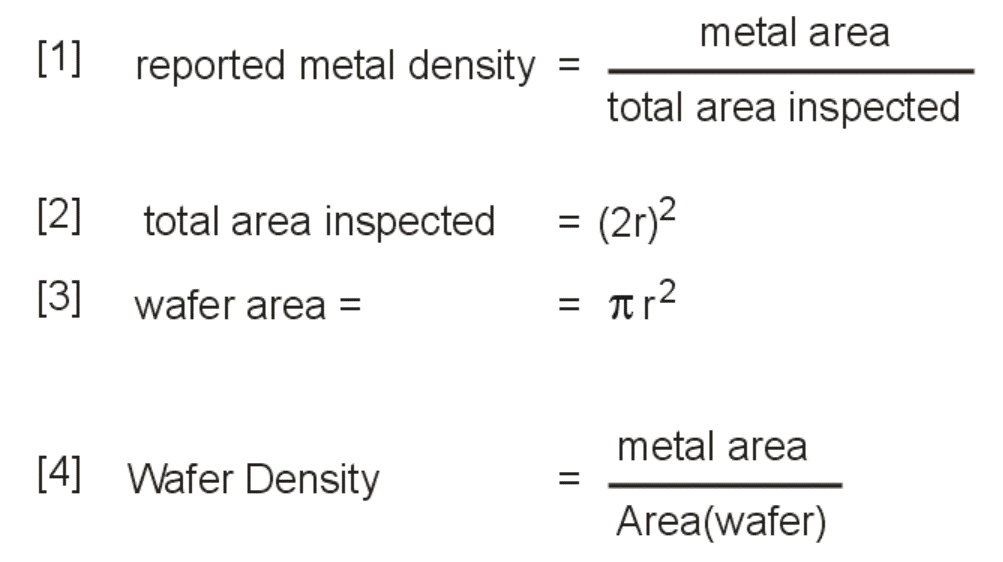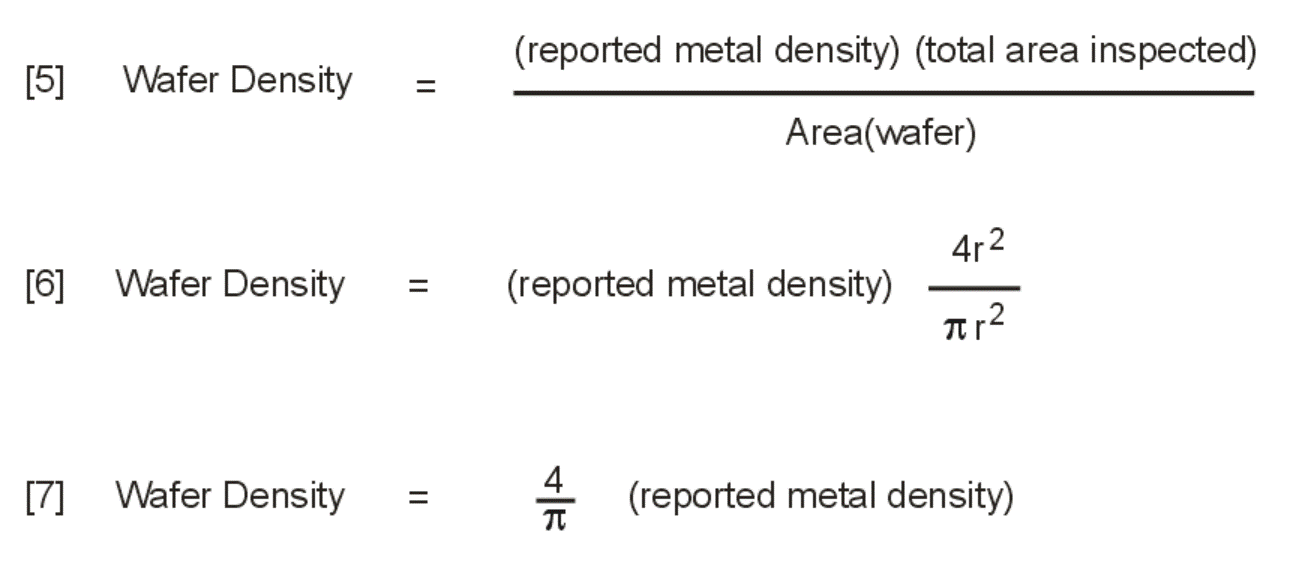# Computing Metal Density of a Round Wafer

In the IC packaging industry, fan-in and fan-out (FOWLP) packages are processed on round substrates. For plating purposes one often wants to know the density of the RDL metal over the entire wafer. The areafill program can only report back density for a rectangular or square region -- not for a round region. So are we unable to get an answer from areafill?

Yes, we can because we know the ratio of the square's area relative to the wafer area and we also know that the corners have no metal at all.Figure 1 - We want to get the metal density over the the round wafer.

The diagram at right shows the relationship between the round wafer and the square bounding box we will pass to areafill as the window over which to do the computation.

We can get an answer using some simple algebra since we know that the density in the corners is always zero.Figure 2 - Relationship between the wafer and the bounding square.

### Definitions

From the definition of density and the geometry shown in Figure 2 we can say:Solving for Wafer Density gives us:### Conclusion

So for the geometry described above (a round wafer bounded by a square region) we can use areafill to compute the density inside the square region and then multiply by 1.27 to get the density of the wafer. This multiplier is independent of the wafer's diameter.